# NCERT Solutions For Class 6 Maths Fractions Exercise 7.4

ncert textbook

## NCERT Solutions For Class 6 Maths Fractions Exercise 7.4

NCERT Solutions for Class 6 Maths Chapter 7 Fractions Ex 7.4

Exercise 7.4

Question 1.
Write shaded portion as fraction. Arrange them in ascending and descending order using correct sign ‘<‘, ‘=’, ‘>’ between the fractions.(c) Show$\frac { 2 }{ 4 }$,$\frac { 4 }{ 6 }$,$\frac { 8 }{ 6 }$and$\frac { 6 }{ 6 }$on the number line. Put appropriate signs between the fractions given.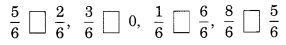Solution:
(a) Total number of divisions = 8
(i) Number of shaded parts = 3
∴ Fraction =$\frac { 3 }{ 8 }$

(ii) Total number of divisions = 8
Number of shaded parts = 6
∴ Fraction =$\frac { 6 }{ 8 }$

(iii) Total number of divisions = 8
Number of shaded parts = 4
∴ Fraction =$\frac { 4 }{ 8 }$

(iv) Total number of divisions = 8
Number of shaded part = 1
∴ Fraction =$\frac { 1 }{ 8 }$
Now the fractions are:$\frac { 3 }{ 8 }$,$\frac { 6 }{ 8 }$,$\frac { 4 }{ 8 }$and$\frac { 1 }{ 8 }$with same denominator.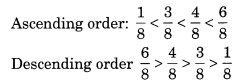(b)(i) Total number of divisions = 9
Number of shaded parts = 8
∴ Fraction =$\frac { 8 }{ 9 }$
(ii) Total number of divisions = 9
Number of shaded parts = 4
∴ Fraction =$\frac { 4 }{ 9 }$
(iii) Total number of divisions = 9
Number of shaded parts = 3
∴ Fraction =$\frac { 3 }{ 9 }$
(iv) Total number of divisions = 9
Number of shaded parts = 6
∴ Fraction =$\frac { 6 }{ 9 }$
∴ Fractions are$\frac { 8 }{ 9 }$,$\frac { 4 }{ 9 }$,$\frac { 3 }{ 9 }$,$\frac { 6 }{ 9 }$with same denominator.Question 2.
Compare the fractions and put an appropriate sign.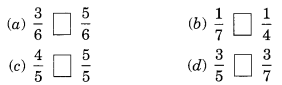Solution:Here, denominators of the two fractions are same and 3 < 5.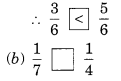Here, numerators of the fractions are same and 7 > 4.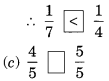Here, denominators of the two fractions are same and 4 < 5.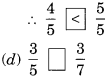Here, numerators of the two fractions are same and 5 < 7.Question 3.
Make five more such pairs and put appropriate signs.
Solution:Question 4.
Look at the figures and write ’<’, or ’>’ ’=’ between the given pairs of fractions.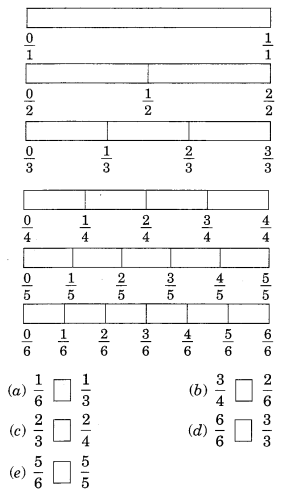Make five more such problems and solve them with your friends
Solution:Make five more such problems yourself and solve them with your friends.

Question 5.
How quickly can you do this? Fill appropriate sign. ‘<‘, ‘=’, ‘>’.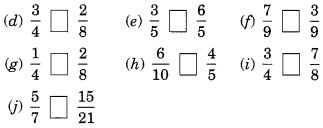Solution: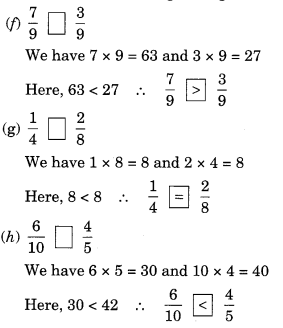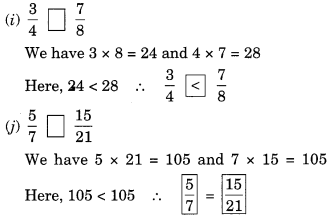Question 6.
The following fractions represent just three different numbers. Separate them into three groups of equivalent fractions, by changing each one to its simplest form.Solution: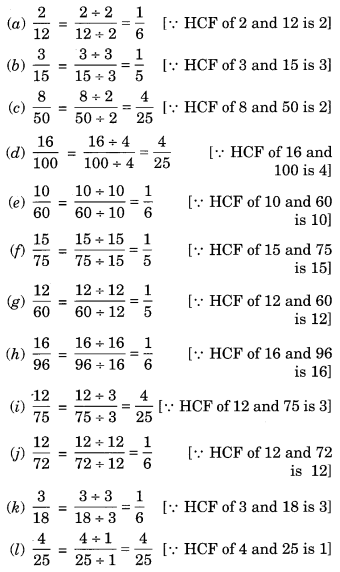Now grouping the above fractions into equivalent fractions, we have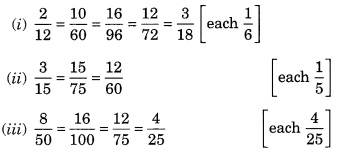Question 7.
Find answers to the following. Write and indicate how you solved them.Solution:By cross-multiplying, we get
5 x 5 = 25 and 4 x 9 = 36
Since 25 ≠ 36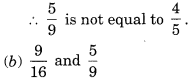By cross-multiplying, we get
9 x 9 = 81 and 16 x 5 =80
Since 81 ≠ 80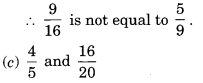By cross-multiplying, we get
4 x 20 = 80 and 5 x 16 = 80
Since 80 = 80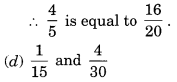By cross-multiplying, we get
1 x 30 = 30 and 4 x 15 = 60Question 8.
Ila read 25 pages of a book containing 100 pages.
Lalita read$\frac { 2 }{ 5 }$of the same book. Who read less?
Solution:
Ila reads 25 pages out of 100 pages.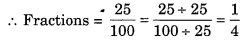Lalita reads$\frac { 2 }{ 5 }$of the same book.
Comparing$\frac { 1 }{ 4 }$and$\frac { 2 }{ 5 }$, we get
1 x 5 = 5 and 2 x 4 = 8
Since 5 < 8$\frac { 1 }{ 4 }$<$\frac { 2 }{ 5 }$

Question 9.
Rafiq exercised for$\frac { 3 }{ 6 }$of an hour, while Rohit exercised for$\frac { 3 }{ 4 }$of an hour. Who exercised for a longer time?
Solution:
Rafiq exercised for$\frac { 3 }{ 6 }$of an hour.
Rohit exercised for$\frac { 3 }{ 4 }$of an hour.
Comparing$\frac { 3 }{ 6 }$and$\frac { 3 }{ 4 }$, we get
3 x 4 = 12 and 3 x 6 = 18
Since 12 < 18$\frac { 3 }{ 4 }$>$\frac { 3 }{ 6 }$
Hence Rohit exercised for longer time.

Question 10.
In a class A of 25 students, 20 passed in first class, in another class B of 30 students, 24 passed in first class. In which class was a greater fraction of students getting first class?
Solution:
In class A, 20 students passed in first class out of 25 students.
∴ Fraction of students getting first classIn class B, 24 students passed in first class out of 30 students.
∴ Fraction of students getting first class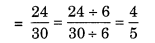Comparing the two fractions, we get$\frac { 4 }{ 5 }$=$\frac { 4 }{ 5 }$
Hence, both the class A and B have the same fractions.